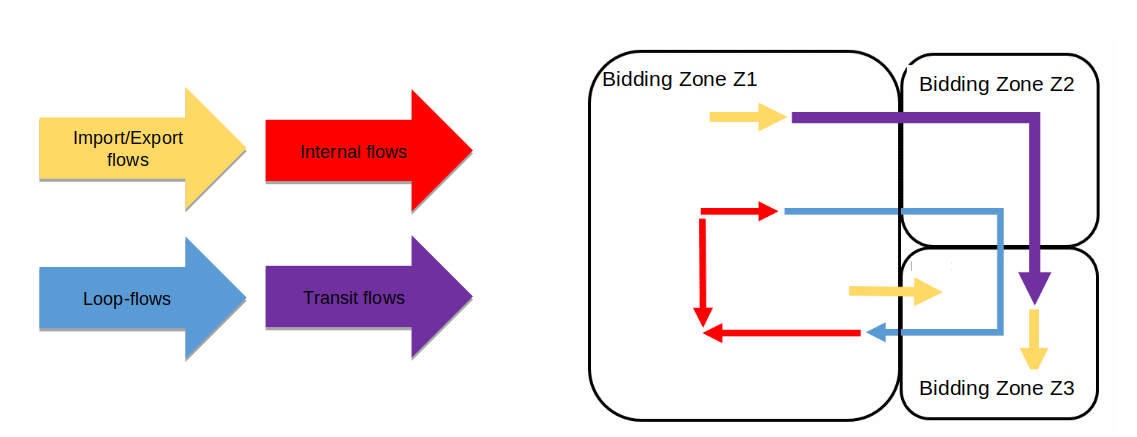# Loop-flows

Contents

### Definition #

When the power flows from the production areas to the consumers, the current physically takes several paths. The flow can be composed of:

• When production and consumers are in the same bidding zone Z:
• internal flows: the current travels through lines that are in Z
• loop-flows: the current travels through lines that are in several bidding zones
• When production and consumers are in different bidding zones Z1 and Z2:
• import/export flows: the current only travels through lines that are in Z1 or Z2
• transit flows: the current travels through lines that are in at least 3 different bidding zonesTherefore, loop-flows are the flows remaining on cross-border lines when no commercial exchanges are present. Loop-flows limit the capacity available for the market.

### Computation #

It is possible to configure the RAO in order to make sure that, during RA optimisation, the loop-flow $$F_{loop-flow}$$ on each cross zonal CNEC does not exceed the maximum between:

• the initial loop-flow $$F_0$$
• the loop-flow threshold $$F_{max_{loop-flow}}$$, provided by TSOs for each of their cross-zonal CNECs

It can be computed using the actual flow and the commercial flow:

$\begin{equation} F_{loop-flow}(c) = F(c) - F_{commercial}(c) \end{equation}$ $\begin{equation} F_{commercial} (c) = \sum_{z \in LFC} PTDF(c,z) * NP(z) \end{equation}$

With:
- LFC, the set of bidding zones for which we compute the commercial flows, set under loop-flow-countries
- NP, the net position of the bidding zone z, read from the ReferenceProgram
- PTDF, the power transfer distribution factor of the bidding zone z on the FlowCnec c, eventually recomputed within the RAO depending on the value of the configuration parameter loop-flow-approximation. The PTDF represents FlowCnec c’s sensitivity to a variation of the net position on the bidding zone Z mapped on the network according to GLSK).

### Implementation #

Loop-flow limitation are modelled in the RAO under the linear problem as constraints for each CNEC $$i$$ forcing the loop-flows within their bounds, see Loop-flow constraints.